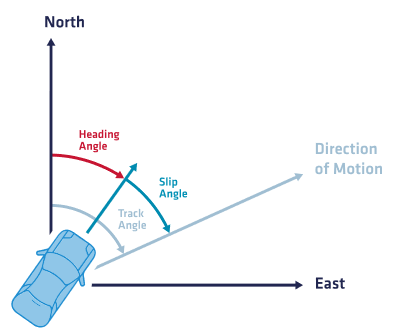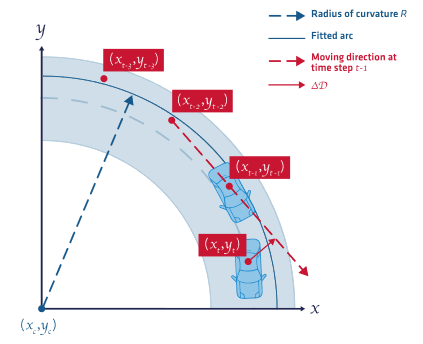Automotive applications sometimes involve specific measurements derived from the standard INS output. The most common outputs are the body velocity, the slip angle and the curvature.

## INS alignment

It is important to understand that automotive specific outputs accuracy are affected by the INS intrinsic performance but also by the mechanical installation within the vehicle. Especially, the INS has to be perfectly aligned with the vehicle frame to ensure valid and consistent measurements.

If for example, the INS is installed with a 1° heading misalignment, the slip angle measurement will be centered around 1° instead of 0°.

Moreover, we consider that the nominal vehicle slip angle and curvature measurements are typically computed at the center of the non steering axle (in general the rear axle).

However it is possible to evaluate these quantities at another location of the vehicle by either installing the INS where you would like the measurements or by using deported monitoring points.When using automotive motion profiles, the INS Kalman Filter estimates and refines any pitch and yaw misalignment to ensure optimal navigation performance. However, this estimated misalignment is only used internally and thus doesn’t impact the data returned by the product.

## Slip angle

The Slip angle is the angle between the course over ground (direction towards which the vehicle is moving) and the heading (direction where the vehicle longitudinal axis is pointing).The following formula is used to compute the slip angle in radians:

$Slip_{angle} = Track_{course} - TrueHeading$

Where Track course is the course over the ground :

$$Track_{course} = tan^{-1} ({V_E \over V_N})$$

Since the course over ground is only defined when the vehicle is moving, this output is only valid in case of velocity above 1 m/s. In addition, if you see a constant slip angle value, it means the INS is not properly aligned (heading angle mainly) with the vehicle frame.vehicle behavior vs Motion profiles

In case the vehicle is expected to experience regular slips/drifts, we recommend the use of motion profiles that do not use automotive constraints assumptions like the Airplane motion profile for example.Slip Angle measurement location

The slip angle measurement is using the 2D velocity information and as such is different at each vehicle location. The most common slip angle measurement is done at the vehicle center of rotation.

### Accuracy

The accuracy of Slip angle output will be directly impacted by several parameters:

• INS velocity accuracy
• Vehicle speed

$$Slip_{acc} = {\sqrt {Heading_{acc}^2 + tan^{-1} {\left({Vel_{acc} \over Speed}\right)^2}}}$$The accuracy is generally provided for a typical speed of 50 Km/h

The following table shows example of expected accuracy at different speed for an ELLIPSE-D in RTK mode (with typical Heading accuracy at 0.1° and velocity at 0.05 m/s):

Speed (km/h)Track Accuracy (°)Slip Accuracy (°)
100.290.30
200.140.17
500.060.11
1000.030.10
2000.010.10

The radius of curvature reflects instantly how sharp the vehicle is turning. In case of constant speed and constant angular velocity, like in a roundabout, the curvature radius will be easily compared to the roundabout radius.The following formula is used to compute the curvature radius in meters:

$$curvature = {\ Vel_{2D} \over w_{Down}}$$

Since the curvature is infinite in case of straight line driving, the value is only computed and valid for angular rate above 1 °/s and velocity above 1 m/s.Curvature measurement location

The curvature measurement is using the 2D velocity information and as such is different at each vehicle location. The most common curvature measurement is done at the vehicle center of rotation.